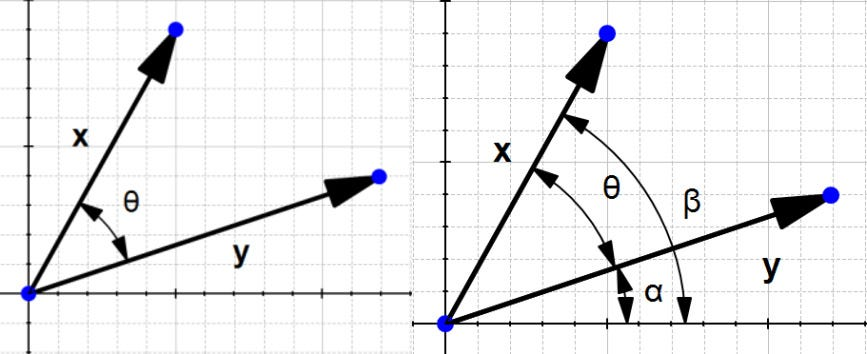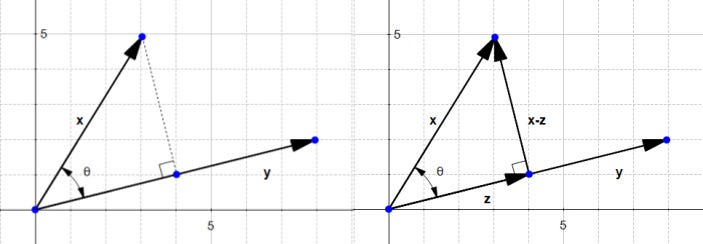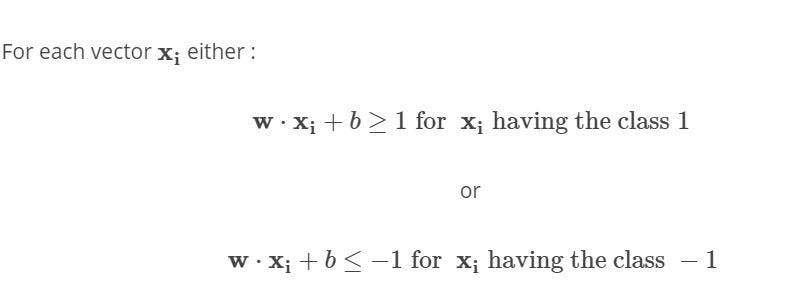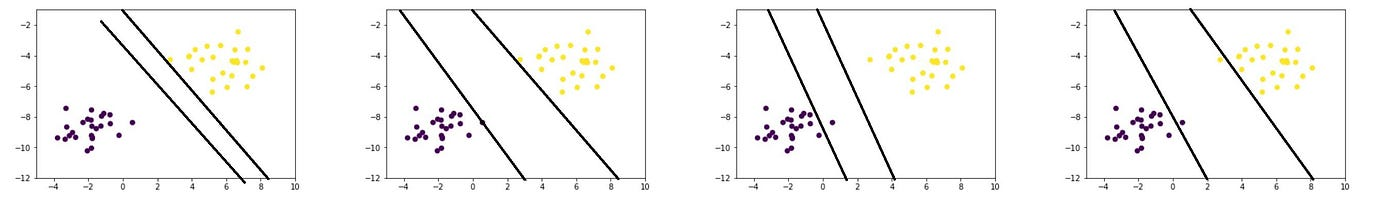# Chapter 3: Support Vector machine with Math.

Last story we talked about Logistic Regression for classification problems, This story I wanna talk about one of the main algorithms in machine learning which is support vector machine. Lets get started!

SVM can be used to solve both classification and regression problems, in this story we talk about classification only(binary classification and linear data).

Before we dive into the story I wanna point this

Why SVM ???

We have couple of other classifiers there, so why do we have to choose SVM over any other ??

Well! It does a pretty good job at classification than others. for example observe the below image.

We have a data-set which is linearly separable from +’s and — ’s , we can separate the data using logistic regression (or other) we may get something like above (which is reasonable).

This is how SVM does

This is a high level view of what SVM does, The yellow dashed line is the line which separates the data (we call this line ‘Decision Boundary’ (Hyperplane) in SVM), The other two lines (also Hyperplanes) help us make the right decision boundary.

so what the heck is hyperplane?

The answer is “a line in more that 3 dimensions” ( in 1-D it’s called a point, in 2-D it’s called a line, in 3-D it’s called a plane, more than 3 - Hyperplane).

How is SVM’s hyperplane different from linear classifiers?

Motivation: Maximize margin: we want to find the classifier whose decision boundary is furthest away from any data point.We can express the separating hyper-plane in terms of the data points that are closest to the boundary. And these points are called support vectors.

We would like to learn the weights that maximize the margin. So we have the hyperplane!

Margin is the distance between the left hyperplane and right hyperplane.

Peroid.

These are couple of examples that I ran SVM (written from scratch) over different data sets.

Okay, you get the idea what SVM does , now Lets talk about how it does???

First I want you to be familiar with the above words so lets complete that

## Vector , its a n dimensional object which has magnitude(length) and direction, it starts from origin(0,0).

The magnitude or length of a vector u(O-A distance) is written ∥ u∥ and is called its norm.

The direction of the vector u is defined by the angle θ with respect to the horizontal axis, and with the angle α with respect to the vertical axis.

Addition and subtraction of Vectors

Dot product of vectorsDot product of x and y(finding the θ )

If we work on a bit we get this ‖x‖‖y‖cos(θ)=x1y1+x2y2

∥x∥∥y∥cos(θ)=x⋅y

The orthogonal projection of a vector.Vector x onto vector y (Left) ,new vector z(right)

Why are we interested by the orthogonal projection ? Well , it allows us to compute the distance between x and the line which goes through y (x-z).

# The SVM hyperplane

The line equation and hyperplane equation — same, its a different way to express the same thing,

It is easier to work on more than two dimensions with the hyperplane notation.

so now we know how to draw a hyperplane with the given dataset,

so what’s next??

We have a data-set , we want to draw a hyper plane something like above (which separates the data well).

How can we find the optimal hyperplane(yellow line) ?

if we maximize the margin(distance) between two hyperplanes then divide by 2 we get the decision boundary.

how do we maximize the margin??

lets take only 2 dimensions, we get the equation for hyper line is

w.x+b=0 which is same as w.x =0 (which has more dimensions)Rules for separating datasetFirst two are following our Rules while others ain’t

if w.x+b=0 then we get the decision boundary

→The yellow dashed line

if w.x+b=1 then we get (+)class hyperplane

for all positive(x) points satisfy this rule (w.x+b ≥1)

if w.x+b=-1 then we get (-)class hyperplane

for all negative(x) points satisfy this rule (w.x+b≤-1)

Observe this picture.

Above both are following our rules so how do I pick the max margin one???

Answer : pick one which has minimum Magnitude of w

This is the final picture designed by me

so either we save the w and b values and keep going or we adjust the parameter (w and b) and keep going. Another optimization problem, SVM.

Adjusting parameters? Sounds like Gradient descent right? Yes!!!

It is a convex optimization problem which surely gives us global minmum value.

Once its optimized we are done!

so what’s next??

Predicting!

Now we give a unknown point and we want to predict whether it belongs to positive class or negative class.

That’s it for this story. hope you enjoyed and learned something.

we just talked about half of SVM only , the other half is about Kernals ( we will talk about it later)

In the next story we will code this algorithm from scratch (without using any ML libraries).

Until then

See ya!

--

--